Inequalities I

Sol A Subtracting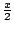and 9 from both sides gives, and then multiplying by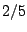givesor. Therefore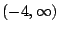is the solution.

Sol B Multiplying both sides out gives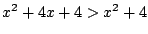, and then subtracting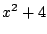from both sides gives. Therefore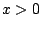, so the solution is given by.

Sol C Solvinggivesor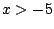, and solving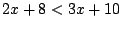givesor. Taking the values ofsatisfying both inequalities gives, sois the solution.

Sol D Solvinggives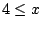or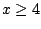, and solvinggives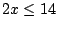or. Taking the values ofsatisfying both inequalities gives, sois the solution.

Sol 1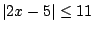iffiffiff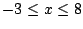, so the solution is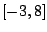.

Sol 2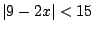iffiff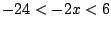iffiff, so the solution is.

Sol 3iff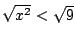iffiff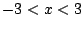, so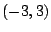is the solution.

Sol 4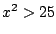iff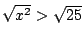iff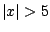iffor, so the solution is.

Sol 5iff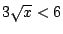iffiffandiff, so the solution is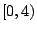.

Sol 6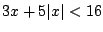can be broken up into the following two cases: a) If, thengives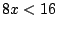or; so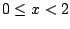.

b) If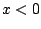, thengivesor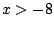; so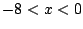.

Combining the solutions for the two cases gives thatis the solution.

Sol 7iffiff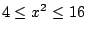iffiffiffor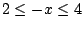ifforiffor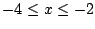, so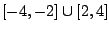is the solution.